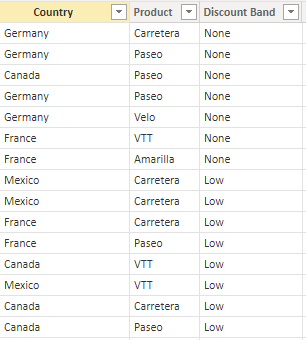cancel
Showing results for
Did you mean:Anonymous
Not applicable

## Store filtered column values in a list, then if column values in list, populate new column.Possible?I have used the above data as an example and I am wondering if it is possible to do the following ?

1) Find all countries for which discount band is low and save this list as a variable

2) Then for all countries in this list, populate a new column with value Y if the country column is in the list.

The pseudocode is as follows:

list = countries where discount band is low

for x in rows

if x[Country] is in list:

populate new column with value Y

END

Is this possible ?

1 ACCEPTED SOLUTIONSuper User

@Anonymous , In case you need a new column havin value of value column, you can have new column like

low value = var _tab = summarize(filter(Table, Table[Discount band] = "Low") , [Country])

return

if([country] in _tab , [Value], blank())Super User

@Anonymous , In case you need a new column havin value of value column, you can have new column like

low value = var _tab = summarize(filter(Table, Table[Discount band] = "Low") , [Country])

return

if([country] in _tab , [Value], blank())